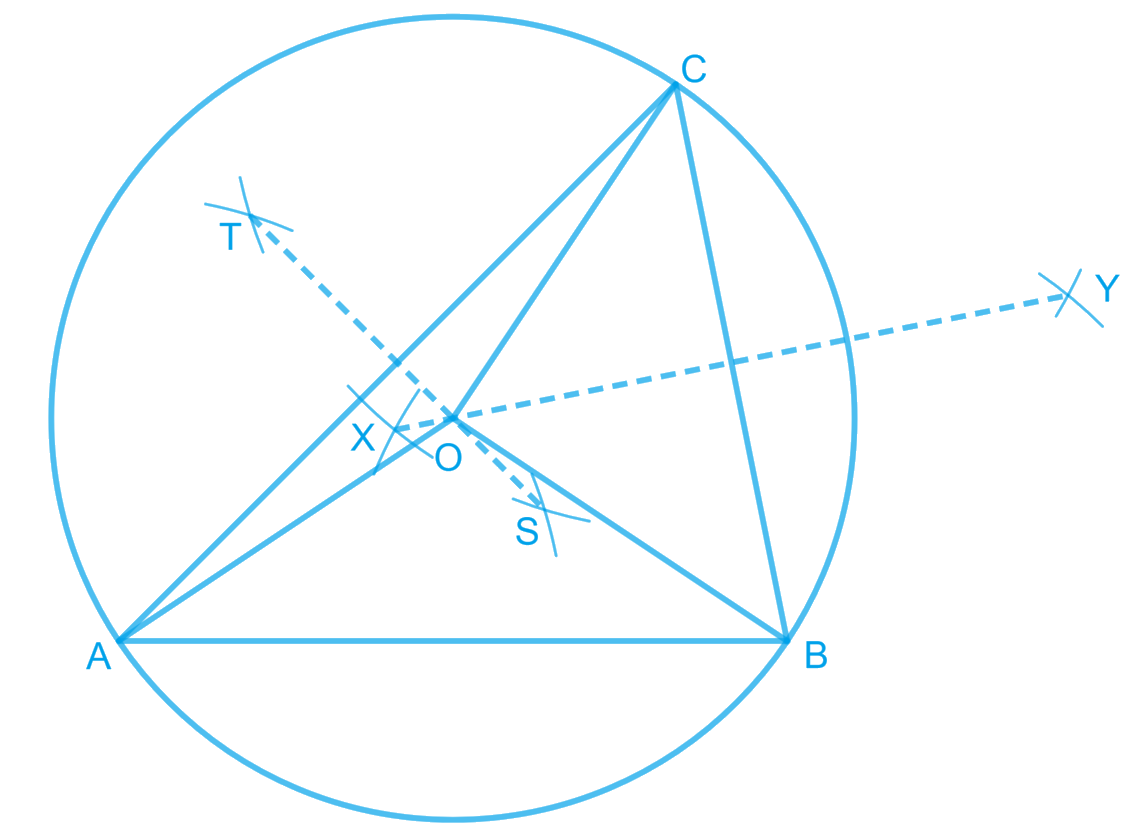In the verge of coronavirus pandemic, we are providing FREE access to our entire Online Curriculum to ensure Learning Doesn't STOP!

# Ex.7.5 Q1 Triangles Solution - NCERT Maths Class 9

Go back to  'Ex.7.5'

## Question

$$ABC$$ is a triangle. Locate a point in the interior of $$ΔABC$$ which is equidistant from all the vertices of $$ΔABC.$$

Video Solution
Triangles
Ex 7.5 | Question 1

## Text Solution

Steps:

Circumcentre of a triangle is always equidistant from all the vertices of that triangle.

Circumcentre is the point where perpendicular bisectors of all the sides of the triangle meet together.In $$\Delta ABC$$, we can find the circumcentre by drawing the perpendicular bisectors of sides $$AB, BC,$$ and $$CA$$ of this triangle. $$O$$ is the point where these bisectors are meeting together. Therefore, $$O$$ is the point which is equidistant from all the vertices of $$\Delta ABC$$.

Video Solution
Triangles
Ex 7.5 | Question 1

Learn from the best math teachers and top your exams

• Live one on one classroom and doubt clearing
• Practice worksheets in and after class for conceptual clarity
• Personalized curriculum to keep up with school